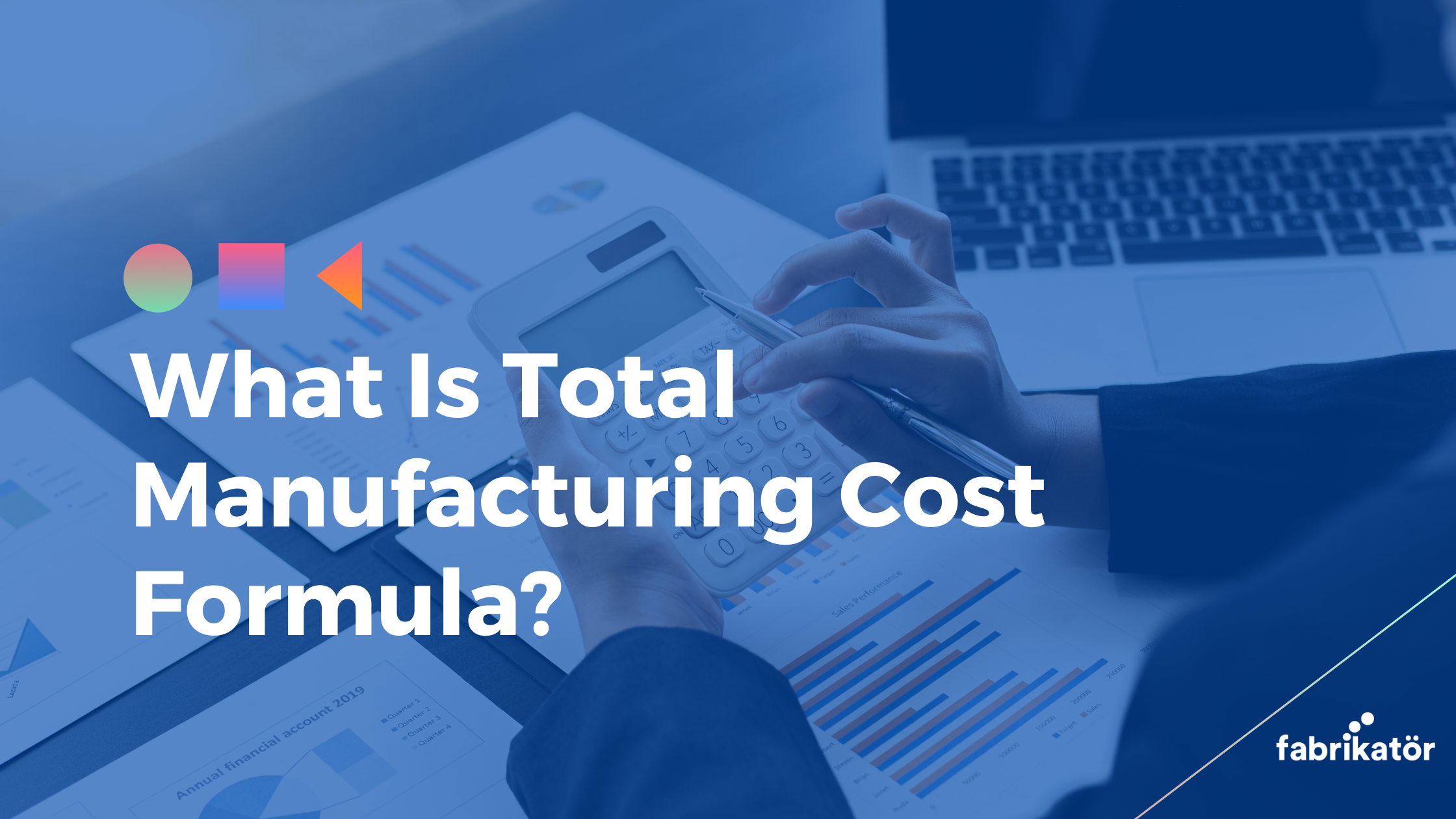# What Is Total Manufacturing Cost Formula?The main purpose of the enterprises is to make profitability permanent and to guarantee the existence of these ways. For this, every activity carried out by the business must be recorded and within a certain plan framework. This becomes even more important for manufacturing companies. Knowing the cost spent on production is vital in terms of calculating the profitability of the business and determining a strategy for the future. However, in many enterprises, the total cost calculation is done incorrectly. In order to make the correct calculation, it is necessary to use the total manufacturing cost formula. A company cannot make a plan for the continuation of its existence without knowing exactly what the cost of the unit it has produced.

## What is Total Manufacturing Cost?

The total manufacturing cost formula refers to the total of all costs incurred for the production of the unit product in the enterprises producing. In the classical calculations, only the method used for the raw material is divided by the number of products produced. However, this is an erroneous calculation method. Because the raw material is not the only cost in production. Some of the issues affecting cost in production are:

• Direct Materials: It expresses the existence of raw materials and production tools required for manufacturing. While the total manufacturing cost formula is calculated, the depreciation amount of the vehicles and machines used in production should be calculated. In other words, the wear rate of the vehicle should be included in the cost.
• Direct Labor: An indispensable part of the production is labor. Although most of the work is done by machines, salaries given to qualified and unqualified personnel who control this process are one of the issues that make up the cost of production.
• Manufacturing (Frim) Overhead: It refers to all the other costs that the company mobilizes and spends for production. All expenses such as the rent of the place used for production, warehouse rent, and electric money are included in this item.

You may also like: How to Calculate Throughput Time

## Formula of total cost of manufacturing

The total manufacturing cost formula is a fairly simple formula. However, it is still necessary to be very careful when calculating this formula manually. A mistake to be made while determining the cost may cause the company to produce at a loss and not notice it.

For the total manufacturing cost formula, the amount of raw material required for 1-day production should be written first. Then, the part of the salaries of the employee or employees working in the production phase, which corresponds to 1 day, should be taken. The 1-day amount of the invoice of the electricity used by the enterprise in production, one-day cost of the workplace or warehouse rental, and the depreciation amounts of the production vehicles should be written one after the other. Then, the amount of product to be obtained should be determined by targeting 1-day production. Finally, the total costs are divided by the number of products produced, and the cost per unit is calculated.

You may also like: How to Reduce Production Costs?

## 2 Examples for total cost of manufacturing

In order to better understand the total manufacturing cost formula, it is necessary to give a few examples. When we consider a company producing cardboard cups, the amount of raw material required for 1-day production is first calculated. Let's accept this value as \$ 1.000. Let's calculate that there are 2 workers working at the production stage and the total costs to the enterprise at 8.000 TL. By dividing this into 30 days, 1-day labor cost is calculated as \$ 266.66.

Let's assume that the amount of 1 day from the electricity bill is 100 TL, the daily depreciation of the production vehicles is \$ 100, and the amount of the daily share of the rental fee is \$ 200. In total, the daily cost of the enterprise is reached from \$ 1666,66 from 1.000 + 266,66 + 100 + 100 + 200 accounts. Let's take the number of cardboard cups produced in one day with the raw material available to the enterprise as 20.000 pieces. In order to find the cost per product, 2.666,66 / 20.000 transactions should be done. As a result, there is 0.13333. In other words, the cost of one produced cardboard cup is \$ 0.133333.

As a different example, consider a factory that produces ready-made water. In this factory, \$ 2.000 for pet bottles in a day, \$ 750 as the daily expense of workers working in production, \$ 150 as the depreciation of production vehicles, \$ 300 for water extraction and filling operations, \$ 400 as transportation fee for delivering the water to dealers, electricity and other expenses Let's say that \$ 250  is spent. In this case, \$ 3.850 is spent from 2,000 + 750 + 150 + 300 + 400 + 250 transactions as a daily cost. However, let's assume that the factory produces 10,000 pet bottles of water daily. So 3.850 / 10,000 is done to find the cost per unit and as a result, the number 0.338 is obtained. In other words, the total cost of this factory for one unit of product is \$ 0.338.